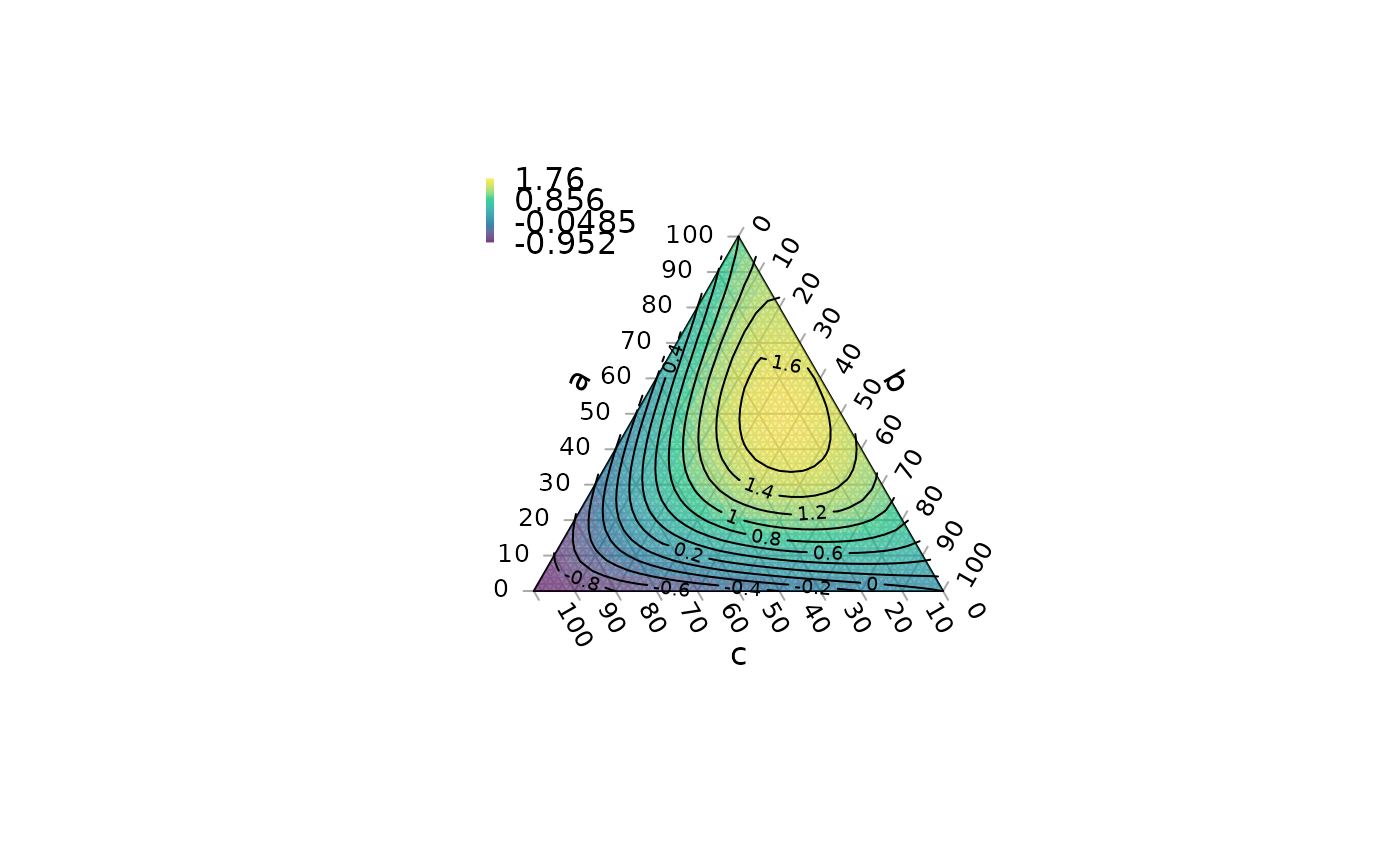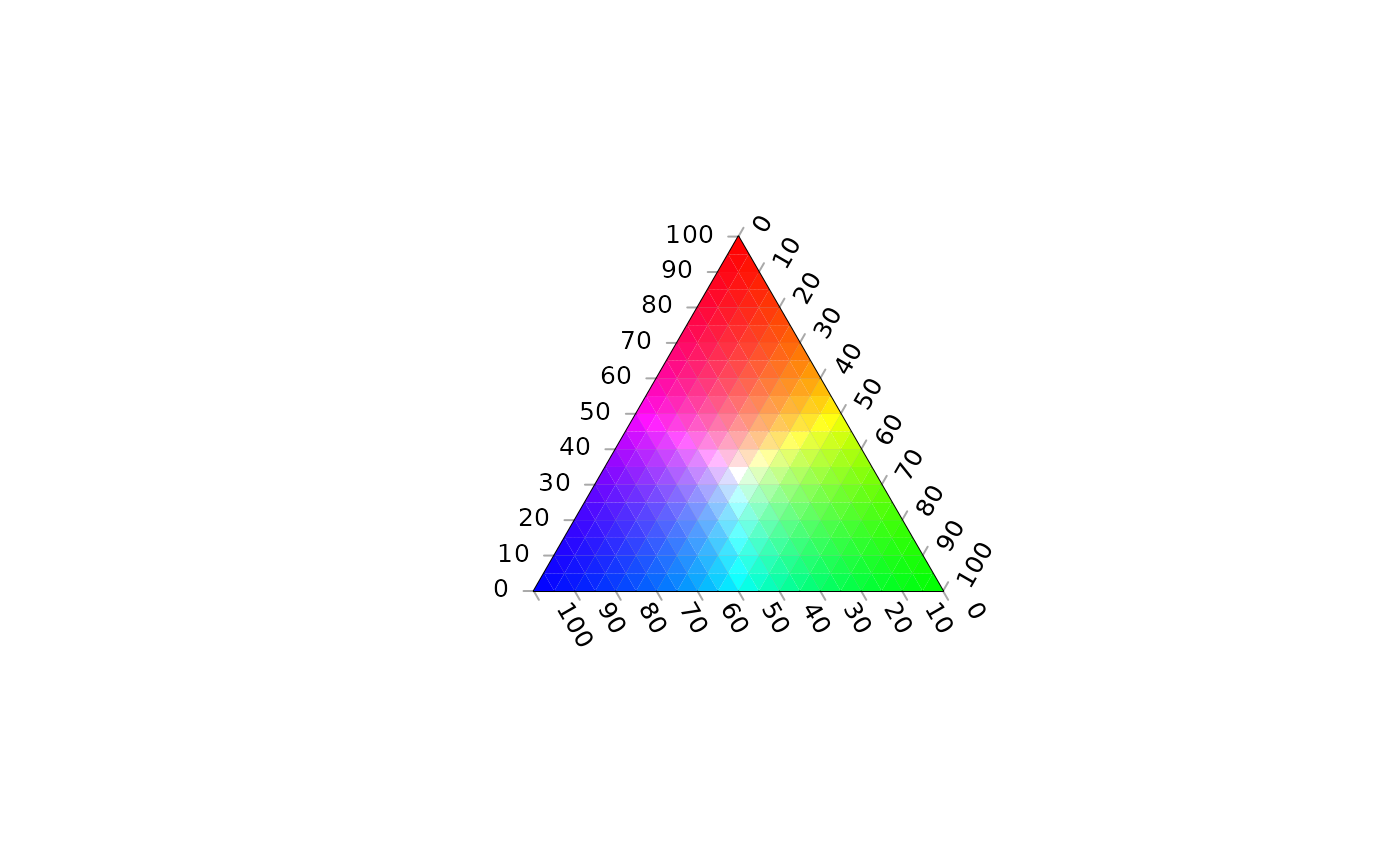Colour a ternary plot according to the output of a function

ColourTernary(
values,
spectrum = viridisLite::viridis(256L, alpha = 0.6),
resolution = sqrt(ncol(values)),
direction = getOption("ternDirection", 1L)
)

ColorTernary(
values,
spectrum = viridisLite::viridis(256L, alpha = 0.6),
resolution = sqrt(ncol(values)),
direction = getOption("ternDirection", 1L)
)

## Arguments

values

Numeric matrix, possibly created using TernaryPointValues(), with four named rows: x, y, cartesian coordinates of each triangle centre; z, value associated with that coordinate; down, triangle direction: 0 = point upwards; 1 = point downwards.

spectrum

Vector of colours to use as a spectrum, or NULL to use values["z", ].

resolution

The number of triangles whose base should lie on the longest axis of the triangle. Higher numbers will result in smaller subdivisions and smoother colour gradients, but at a computational cost.

direction

(optional) Integer specifying the direction that the current ternary plot should point: 1, up; 2, right; 3, down; 4, left.

Other contour plotting functions: TernaryContour(), TernaryDensityContour(), TernaryPointValues()

Other functions for colouring and shading: TernaryTiles()

## Examples

TernaryPlot(alab = "a", blab = "b", clab = "c")

FunctionToContour <- function (a, b, c) {
a - c + (4 * a * b) + (27 * a * b * c)
}

values <- TernaryPointValues(FunctionToContour, resolution = 24L)
ColourTernary(values)
TernaryContour(FunctionToContour, resolution = 36L)TernaryPlot()
values <- TernaryPointValues(rgb, resolution = 20)
ColourTernary(values, spectrum = NULL)# Create a helper function to place white centrally:
rgbWhite <- function (r, g, b) {
highest <- apply(rbind(r, g, b), 2L, max)
rgb(r/highest, g/highest, b/highest)
}

TernaryPlot()
values <- TernaryPointValues(rgbWhite, resolution = 20)
ColourTernary(values, spectrum = NULL)